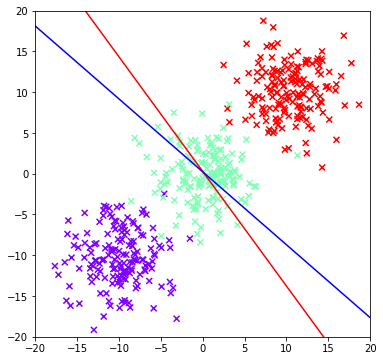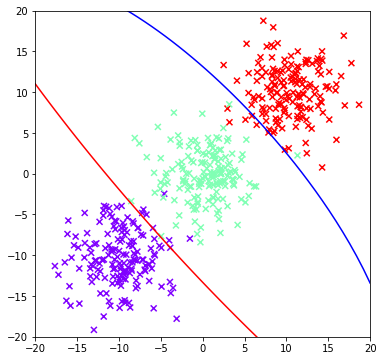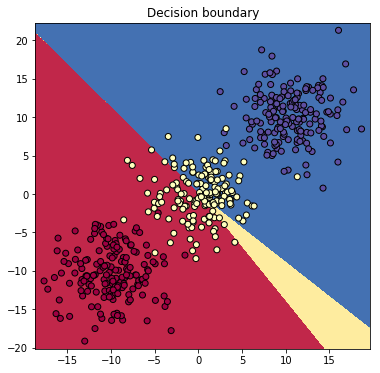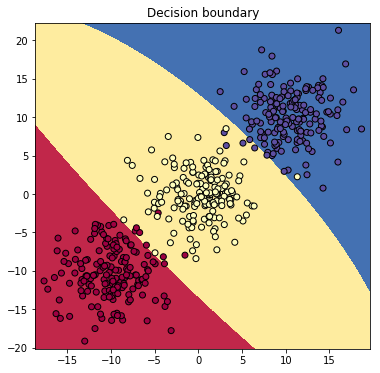# Classification by Regression¶

April 18, 2018 $\newcommand{\bs}{\boldsymbol}$ $\newcommand{\argmin}{\underset{\bs{#1}}{\text{arg min}}\,}$ $\newcommand{\argmax}{\underset{\bs{#1}}{\text{arg max}}\,}$ $\newcommand{\tr}{^{\top}}$ $\newcommand{\norm}{\left|\left|\,#1\,\right|\right|}$ $\newcommand{\given}{\,|\,}$ $\newcommand{\st}{\,\big|\,}$ $\newcommand{\E}{\mathbb{E}\left[#1\right]}$ $\newcommand{\P}{\mathbb{P}\left(#1\right)}$ $\newcommand{\abs}{\left|#1\right|}$ $\newcommand{\blue}{{\color{blue} {#1}}}$ $\newcommand{\red}{{\color{red} {#1}}}$ $\newcommand{\orange}{{\color{orange} {#1}}}$ $\newcommand{\pfrac}{\frac{\partial #1}{\partial #2}}$

Let $x$ be a data vector, and define a classifier $C(x)$ that takes on values in a set of $K$ classes $\mathcal{C}=\{1,2,...,K\}$. For each of the $K$ classes, we define a discriminant function $\delta_k(x)$, so that

$$C(x) = \argmax{k \in \mathcal{C}}{\delta_k(x)}. \tag{1}$$

One popular discriminant function is the posterior probability $\delta_k(x) = P(C=k \given x)$. In this case (1) makes the most sense: we classify to a class that has the highest probability to occur. The decision boundary between any two classes $c_i$ and $c_j$ is the set

$$D = \{x \st \delta_i(x) = \delta_j(x)\}. \tag{2}$$

On $D$, the classifier is said to be indifferent between classifying to class $i$ and to class $j$. If some monotone transformation of the discriminant function is linear, then the decision boundary is linear, and we refer to $C$ as a linear classifier. Common monotone transformations are the log function and the logit function. If we associate a response variable $Y_k$ to each class $k$, and imbue to it a probabilistic model, we will have $K$ generalized linear models, so the discriminant function $\delta_k(x)$ can be interpreted as the conditional expectation $E(Y_k \given x)$. This idea is central in our discussion.

Mathematical prowess does not come from memorizing facts, but from learning the tiny details that help build up the final result and apply them creatively in other settings.

As in the previous section, assume $\mathcal{C}=\{1,...,K\}$ and associate with each class $k$ an indicator variable $Y_k = I(C=k)$. Define $Y\tr = (Y_1,...,Y_K)'$ as a indicator response vector whose terms are zeros with only a single 1. Stack these indicator response vectors as the rows of a $n\times K$ indicator response matrix $\bs{Y}$:

$$\bs{Y} = \begin{bmatrix} Y_1^{(1)} & Y_2^{(1)} & \cdots & Y_K^{(1)} \\ Y_1^{(2)} & Y_2^{(2)} & \cdots & Y_K^{(2)} \\ \vdots & \vdots & \ddots & \vdots \\ Y_1^{(n)} & Y_2^{(1)} & \cdots & Y_K^{(n)} \end{bmatrix}.$$

Given the covariate vectors $x_1,...,x_p$, define the $n\times (p+1)$ design matrix in the usual manner: $X=[\bs{1}, x_1,...,x_p]$. We fit $K$ linear regression models simultaneously in a single equation:

$$\bs{Y} = X\bs{B} + \bs{E},$$

where $\bs{B} = [\beta_1,...,\beta_K]$ is the $p\times K$ coefficient matrix, and $\bs{E}=[\epsilon_1,...,\epsilon_K]$ where each $\epsilon_k \sim \mathcal{N}(\bs{0}, \sigma^2I)$. The solution is easily seen as

$$\hat{\bs{Y}} = X\hat{\bs{B}} := X(X\tr X)^{-1} X\tr \bs{Y}. \tag{3}$$

The columns of the fitted coefficient matrix $\hat{\bs{B}}=[\hat{\beta}_1, \hat{\beta}_2,...,\hat{\beta}_K]$ are the fitted regression coefficients for each of the $K$ classes. For a new observation $x$ and a class $k$, the discriminant function is given by

$$\delta_k(x) = (1, x\tr)\hat{\beta}_k.$$

Hence, the decision boundary between class $i$ and class $j$ is

$$(1, x\tr)(\hat{\beta}_i - \hat{\beta}_j) = 0, \tag{4}$$

which is indeed a linear function in $x$. The fitted value is a vector of $K$ components,

$$\hat{f}(x)\tr = (1, x\tr)\hat{\bs{B}} = (\delta_1(x),...,\delta_K(x)),$$

and the classification is made by

$$\hat{C}(x) = \argmax{k\in \mathcal{C}}{\hat{f}_k(x)}.$$

So why does this method make sense? Under the normal model, we assume that

$$Y_k\given x \sim N(\delta_k(x), \sigma^2),$$

but in reality $Y_k$ only takes on values $0$ and $1$, so its conditional expectation is also a probability: $E(Y_k \given x) = P(C=k \given x)$. This shows that the discriminant function $\delta_k(x)$ approximates but is not exactly equal to this probability. (Note that if we assume a Bernoulli distribution for $Y_k$, this becomes the softmax regression.) However, we do have a nice property:

Property 1   If the design matrix $X$ contains a column of $\bs{1}$, then the sum of the fitted values is equal to 1:

$$\sum_{k\in \mathcal{C}} \hat{f}_k(x)=1.$$

Proof   Start with Equation (3), and use the fact that each row of $\bs{Y}$ sum to 1:

\begin{aligned} \sum_{k\in \mathcal{C}} \hat{f}_k(x) &= (1, x\tr)\hat{\bs{B}}\bs{1}\\ &= (1, x\tr)(X\tr X)^{-1}X\bs{Y}\bs{1} \\ &= (1, x\tr)(X\tr X)^{-1}X\bs{1} \\ &= (1, x\tr)\hat{\beta} \end{aligned}

where $\hat{\beta}$ is the least squares solution from regressing the covariates (including the $\bs{1}$ vector for intercept) on the $\bs{1}$ vector. The final equality holds because $\bs{1}$ is already in the column space, so the linear combination that produces the projected vector is

$$\hat{\beta}=(1,0,...,0)',$$

whish shows that $(1, x\tr)\hat{\beta}=1$ for any $x$. $\blacksquare$

An issue with the regression approach is that the values of the discriminant functions can be either negative or greater than 1; in that case, we lose the probabilistic interpretation. Another serious issue is that it tends to mask some classes if the number of classes $K\geq 3$. This is illustrated in the following example.

In :
import numpy as np
import matplotlib.pyplot as plt
from sklearn.datasets import make_blobs

In :
def encode_indicator_matrix(y):
'''
y is a vector of dimension (n,)
return an indicator matrix of size (n,p)
'''
p = np.unique(y).shape
n = y.shape
I = np.eye(p)
Z = [I[y[i],:] for i in range(n)]
return np.array(Z)

In :
# generate data
centers = np.array([[-10, -10], [0, 0], [10, 10]])
data, label = make_blobs(n_samples=500, centers=centers,
n_features=2, cluster_std=3, random_state=25)

Y = encode_indicator_matrix(label) # indicator response matrix
X = np.insert(data, 0, 1, axis=1) # design matrix
B = np.linalg.inv(X.T@X)@X.T@Y # coefficient matrix

# decision boundaries [see Equation (4)]
C1 = (B[:,0] - B[:,1])
a1 = (B[:,0] - B[:,1])
b1 = (B[:,0] - B[:,1])

C2 = (B[:,1] - B[:,2])
a2 = (B[:,1] - B[:,2])
b2 = (B[:,1] - B[:,2])

x = np.linspace(-20, 20, 50)
y1 = -a1/b1*x - C1/b1
y2 = -a2/b2*x - C2/b2

fig = plt.figure(figsize=(6, 6))
plt.scatter(data[:,0], data[:,1], c=label, cmap='rainbow', marker='x')
plt.plot(x, y1, c='red')
plt.plot(x, y2, c='blue')
plt.xlim([-20, 20])
plt.ylim([-20, 20])
plt.show()From the figure above, the decision boundary between class 0 and class 1 (red line) and the decision boundary between class 1 and class 2 (blue line) are very close to each other. This means that the classifier will predict mostly 0's and 2's, so class 2 is masked. Luckily, the issue can be partially solved if we include higher order polynomial terms ($x_1^2, x_2^2, x_1x_2,...$) in our regression model. In general if $K\geq 3$, we require a polynomial regression of degree at least $K-1$ to unmask all classes. The results of a quadratic regression is shown below.

In :
X_aug = np.c_[X, X[:,1]**2, X[:,2]**2, X[:,1]*X[:,2]] # new design matrix
B_aug = np.linalg.inv(X_aug.T@X_aug)@X_aug.T@Y # coefficient matrix

# decision boundaries
C1 = (B_aug[:,0] - B_aug[:,1])
a1 = (B_aug[:,0] - B_aug[:,1])
b1 = (B_aug[:,0] - B_aug[:,1])
c1 = (B_aug[:,0] - B_aug[:,1])
d1 = (B_aug[:,0] - B_aug[:,1])
e1 = (B_aug[:,0] - B_aug[:,1])

C2 = (B_aug[:,1] - B_aug[:,2])
a2 = (B_aug[:,1] - B_aug[:,2])
b2 = (B_aug[:,1] - B_aug[:,2])
c2 = (B_aug[:,1] - B_aug[:,2])
d2 = (B_aug[:,1] - B_aug[:,2])
e2 = (B_aug[:,1] - B_aug[:,2])

# decision boundaries has the following equation:
# a1x1 + b1x2 + c1x1^2 + d1x2^2 + e1x1x2 + C1 = 0

x = np.linspace(-20, 20, 50)

def boundary(x, C, a, b, c, d, e):
y_min, y_max = [], []
for i in x:
roots = np.roots([d, e*i+b, a*i+c*i**2+C])
y_min.append(min(roots))
y_max.append(max(roots))
return y_min, y_max

y1, _ = boundary(x, C1, a1, b1, c1, d1, e1)
_, y2 = boundary(x, C2, a2, b2, c2, d2, e2)

fig = plt.figure(figsize=(6, 6))
plt.scatter(data[:,0], data[:,1], c=label, cmap='rainbow', marker='x')
plt.plot(x, y1, c='red')
plt.plot(x, y2, c='blue')
plt.xlim([-20, 20])
plt.ylim([-20, 20])
plt.show()The quadratic regression solved our problem. We can visualize the classification region more clearly as below.

In :
# classify function
def classify(new_data, B):
return np.argmax(new_data@B, 1)

# Plot decision boundary of 2D classification problem
def plot_decision_boundary(data, label, B, degree=1):
plt.figure(figsize=[6, 6])
x_min, x_max = data[:, 0].min()-1, data[:, 0].max()+1
y_min, y_max = data[:, 1].min()-1, data[:, 1].max()+1
xx, yy = np.meshgrid(np.arange(x_min, x_max, 0.02), np.arange(y_min, y_max, 0.02))
xr, yr = xx.ravel(), yy.ravel()
if degree==1:
new_data = np.c_[np.ones(len(xr)), xr, yr]
else:
new_data = np.c_[np.ones(len(xr)), xr, yr, xr**2, yr**2, xr*yr]
Z = classify(new_data, B)
Z = Z.reshape(xx.shape)
plt.contourf(xx, yy, Z, cmap=plt.cm.Spectral)
plt.scatter(data[:, 0], data[:, 1], c=label, cmap=plt.cm.Spectral, edgecolors='k')
plt.title('Decision boundary')

In :
plot_decision_boundary(data, label, B)In :
plot_decision_boundary(data, label, B_aug, degree=2)In practice, high order polynomial regression is undesirable. If the number of classes is large and the number of predictors is small, we need alternative methods such as linear discriminant analysis or softmax regression.